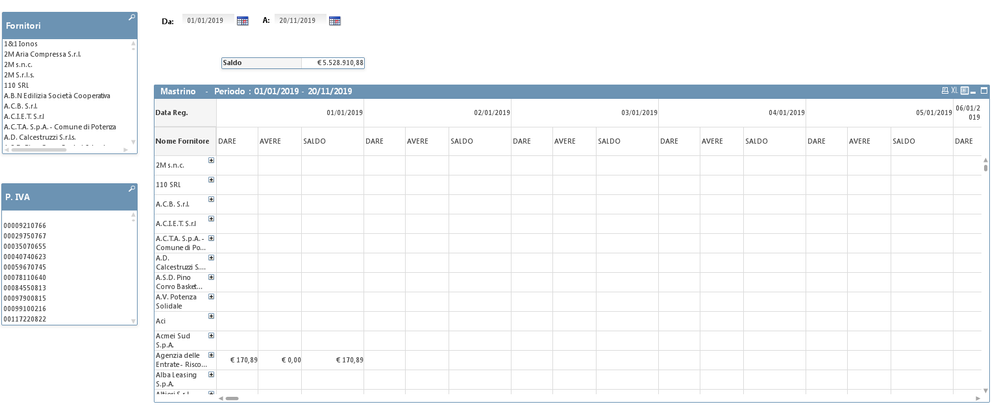# New to QlikView

Discussion board where members can get started with QlikView.

Announcements
Leverage your QlikView investment to modernize BI – see how! Join Group
cancel
Showing results for
Did you mean:
HighlightedContributor III

## Pivot Table dimension - columns of payment date amount by date

I have the following pivot table (see attachment - "Tabella pivot.jpg") with two dimensions and an expression:1) Dimensions: supplier and payment due date

2) Expression:

SUM({<[% Master Calendar Date Num]={">=\$(=\$(vInizioPC))<=\$(=\$(vFinePC))"}, Anno=,Mese=,Giorno=>}[# Imponibile Pagamento])

I would like to have a pivot table with only one dimension (supplier) and the expression must give me the payment amounts for a single date in a column as the attached image (Requested pivot table).

Labels (5)

• ### sum dimension

2 Solutions

Accepted Solutions
HighlightedMVP

## Re: Pivot Table dimension - columns of payment date amount by date

Did you try to drag the date column to the right top corner of the chart? This should let you pivot the Date dimension

HighlightedMVP

## Re: Pivot Table dimension - columns of payment date amount by date

May be you need this?

``````(
RangeSum(Above(TOTAL SUM({<[Master Calendar Date Calc]={">=\$(=\$(vInizioPC))<=\$(=\$(vFinePC))"}, Anno=,Mese=,Giorno=>}[# Importo Fattura Iva]),0,RowNo(TOTAL)))

-

RangeSum(Above(TOTAL SUM({<[Master Calendar Date Calc]={">=\$(=\$(vInizioPC))<=\$(=\$(vFinePC))"}, Anno=,Mese=,Giorno=>}[# Importo Fattura Pagamenti]),0,RowNo(TOTAL)))

)``````
13 Replies
HighlightedMVP

## Re: Pivot Table dimension - columns of payment date amount by date

Did you try to drag the date column to the right top corner of the chart? This should let you pivot the Date dimension

HighlightedContributor III

## Re: Pivot Table dimension - columns of payment date amount by date

thank you very much. If you can give me a hand I would be grateful for another discussion.

https://community.qlik.com/t5/QlikView-Creating-Analytics/Cumulative-Calculations-in-QlikView-Expres...

HighlightedMVP

## Re: Pivot Table dimension - columns of payment date amount by date

I am unable to open your app for some reason.

HighlightedContributor III

## Re: Pivot Table dimension - columns of payment date amount by date

HighlightedMVP

## Re: Pivot Table dimension - columns of payment date amount by date

I can download the qvw file, but I am unable to open it... the qvw window just hangs on me

HighlightedContributor III

## Re: Pivot Table dimension - columns of payment date amount by date

ok try this.

thanks

HighlightedMVP

## Re: Pivot Table dimension - columns of payment date amount by date

May be thisHighlightedContributor III

## Re: Pivot Table dimension - columns of payment date amount by date

No I'm talking about the other sheet; where in another post I asked for the cumulative difference of two columns.

The cumulative difference only works with one dimension if you expand the data in the table no.

The formula used is:

(
RangeSum(Above(SUM({<[Master Calendar Date Calc]={">=\$(=\$(vInizioPC))<=\$(=\$(vFinePC))"}, Anno=,Mese=,Giorno=>}[# Importo Fattura Iva]),0,RowNo()))

-

RangeSum(Above(SUM({<[Master Calendar Date Calc]={">=\$(=\$(vInizioPC))<=\$(=\$(vFinePC))"}, Anno=,Mese=,Giorno=>}[# Importo Fattura Pagamenti]),0,RowNo()))

)Thanks

HighlightedMVP

## Re: Pivot Table dimension - columns of payment date amount by date

May be you need this?

``````(
RangeSum(Above(TOTAL SUM({<[Master Calendar Date Calc]={">=\$(=\$(vInizioPC))<=\$(=\$(vFinePC))"}, Anno=,Mese=,Giorno=>}[# Importo Fattura Iva]),0,RowNo(TOTAL)))

-

RangeSum(Above(TOTAL SUM({<[Master Calendar Date Calc]={">=\$(=\$(vInizioPC))<=\$(=\$(vFinePC))"}, Anno=,Mese=,Giorno=>}[# Importo Fattura Pagamenti]),0,RowNo(TOTAL)))

)``````